graph_graphviz - CSDN
• 核心图数据结构 class tf.Graph 数据流图 class tf.Operation class tf.Tensor 张量类型 class tf.DType tf.as_dtype（type_value） 实用功能 tf.device（DEV） tf.name_scope（name） tf.control_dependencies...
构建图

核心图数据结构 class tf.Graph 数据流图
class tf.Operation class tf.Tensor 张量类型 class tf.DType tf.as_dtype（type_value） 实用功能 tf.device（DEV） tf.name_scope（name） tf.control_dependencies（control_inputs） tf.convert_to_tensor（value，dtype = None，name = None） tf.get_default_graph（） tf.import_graph_def（graph_def，input_map = None，return_elements = None，name = None，op_dict = None） 图集合 tf.add_to_collection(name, value) tf.get_collection（key，scope = None） class tf.GraphKeys 定义新的业务 class tf.RegisterGradient tf.NoGradient（op_type） class tf.RegisterShape class tf.TensorShape class tf.Dimension tf.op_scope（values，name，default_name） tf.get_seed（op_seed） 用于构建TensorFlow图的类和函数。

核心图数据结构

用于构建TensorFlow图的类和函数。

核心图数据结构

class tf.Graph

TensorFlow计算，表示为dataflow graph。

图表包含一组操作对象，表示计算单位; 和Tensor对象一样，表示在操作之间流动的数据单位。

始终默认注册为Graph，并可通过调用tf.get_default_graph（）来访问。 要将operation添加到默认Graph， 只需调用其中一个函数定义新的operation：

In :

import tensorflow as tf
c = tf.constant(4.0)
assert c.graph is tf.get_default_graph()

In :

c.graph

Out:

<tensorflow.python.framework.ops.Graph at 0x2589729c8d0>

另一种典型的用法涉及Graph.as_default()上下文管理器，它在上下文的生存期内覆盖当前的默认图形：

In :

g = tf.Graph()
with g.as_default():
# Define operations and tensors in g.
c = tf.constant(30.0)
assert c.graph is g

重要注意：对于图的构造，这个类不是线程安全的。所有操作都应该从单个线程创建，或者必须提供外部同步。除非另有规定，否则所有方法都不是线程安全的.

tf.Graph.init()

Creates a new, empty Graph.

tf.Graph.as_default()

返回使此Graph成为默认图形的上下文管理器。

如果要在同一进程中创建多个图，则应使用此方法。 为方便起见，提供了一个全局默认图，如果您没有明确创建新图，则所有操作都将添加到此图中。 使用此方法with关键字指定应将在块范围内创建的操作添加到此图中。

默认图是当前线程的属性。 如果您创建一个新线程，并希望在该线程中使用默认图形，则必须在该线程的函数中显式添加 with g.as_default（）:.

以下代码示例是等效的：

In :

# 1. Using Graph.as_default():
g = tf.Graph()
with g.as_default():
c = tf.constant(5.0)
assert c.graph is g

# 2. Constructing and making default:
with tf.Graph().as_default() as g:
c = tf.constant(5.0)
assert c.graph is g
# Returns:
# A context manager for using this graph as the default graph.

tf.Graph.as_graph_def(from_version=None)

Returns a serialized GraphDef representation of this graph.

The serialized GraphDef can be imported into another Graph (using import_graph_def()) or used with the C++ Session API.

Args: from_version: Optional. If this is set, returns a GraphDef containing only the nodes that were added to this graph since its version property had the given value. Returns: A GraphDef protocol buffer.

tf.Graph.finalize()

最后确定这个图表，使它成为只读的。 在调用g.finalize()之后，不能向g添加任何新的操作。此方法用于确保图形在多个线程之间共享时不会添加任何操作，例如在使用QueueRunner时。

tf.Graph.control_dependencies(control_inputs)

Returns a context manager that specifies control dependencies.

Use with the with keyword to specify that all operations constructed within the context should have control dependencies on control_inputs. For example:

d and e will only run after a, b, and c have executed.

with g.control_dependencies([a, b, c]):

d = ...
e = ...

Multiple calls to control_dependencies() can be nested, and in that case a new Operation will have control dependencies on the union of control_inputs from all active contexts.

with g.control_dependencies([a, b]):

Ops declared here run after a and b.

with g.control_dependencies([c, d]):

# Ops declared here run after a, b, c, and d.


N.B. The control dependencies context applies only to ops that are constructed within the context. Merely using an op or tensor in the context does not add a control dependency. The following example illustrates this point: 注： 控制依赖关系上下文仅适用于在上下文中构造的操作。 仅在上下文中使用op或tensor不会添加控件依赖项。 以下示例说明了这一点：

In [ ]:

# WRONG
def my_func(pred, tensor):
t = tf.matmul(tensor, tensor)
with tf.control_dependencies([pred]):
# The matmul op is created outside the context, so no control
return t

# RIGHT
def my_func(pred, tensor):
with tf.control_dependencies([pred]):
# The matmul op is created in the context, so a control dependency
return tf.matmul(tensor, tensor)

Args: control_inputs: A list of Operation or Tensor objects, which must be executed or computed before running the operations defined in the context. Returns: A context manager that specifies control dependencies for all operations constructed within the context.

Raises: TypeError: If control_inputs is not a list of Operation or Tensor objects.

-

tf.Graph.device(device_name_or_function)

Returns a context manager that specifies the default device to use.

device_name_or_function参数可以是设备名称字符串，设备函数或None：

如果它是设备名称字符串，则在此上下文中构造的所有操作都将分配给具有该名称的设备。 如果它是一个函数，它将被视为从操作对象到设备名称字符串的函数，并在每次创建新操作时调用。 操作将以返回的名称分配给设备。 如果为None，则清除默认设备。 For example: with g.device('/gpu:0'):

All operations constructed in this context will be placed

on GPU 0.

with g.device(None):

# All operations constructed in this context will have no
# assigned device.



Defines a function from Operation to device string.

def matmul_on_gpu(n): if n.type == "MatMul": return "/gpu:0" else: return "/cpu:0"

with g.device(matmul_on_gpu):

All operations of type "MatMul" constructed in this context

will be placed on GPU 0; all other operations will be placed

on CPU 0.

In [ ]:

Args:
device_name_or_function: The device name or function to use in the context.
Returns:
A context manager that specifies the default device to use for newly created ops.

tf.Graph.name_scope(name)
Returns a context manager that creates hierarchical names for operations.

A graph maintains a stack of name scopes. A with name_scope(...): statement pushes a new name onto the stack for the lifetime of the context.

The name argument will be interpreted as follows:

A string (not ending with '/') will create a new name scope, in which name is appended to the prefix of all operations created in the context. If name has been used before, it will be made unique by calling self.unique_name(name).
A scope previously captured from a with g.name_scope(...) as scope: statement will be treated as an "absolute" name scope, which makes it possible to re-enter existing scopes.
A value of None or the empty string will reset the current name scope to the top-level (empty) name scope.
For example:

with tf.Graph().as_default() as g:
c = tf.constant(5.0, name="c")
assert c_1.name == "c"
c_1 = tf.constant(6.0, name="c")
assert c_1.name == "c_1"

# Creates a scope called "nested"
with g.name_scope("nested") as scope:
nested_c = tf.constant(10.0, name="c")
assert nested_c.name == "nested/c"

# Creates a nested scope called "inner".
with g.name_scope("inner"):
nested_inner_c = tf.constant(20.0, name="c")
assert nested_inner_c.name == "nested/inner/c"

# Create a nested scope called "inner_1".
with g.name_scope("inner"):
nested_inner_1_c = tf.constant(30.0, name="c")
assert nested_inner_1_c.name == "nested/inner_1/c"

# Treats scope as an absolute name scope, and
# switches to the "nested/" scope.
with g.name_scope(scope):
nested_d = tf.constant(40.0, name="d")
assert nested_d.name == "nested/d"

with g.name_scope(""):
e = tf.constant(50.0, name="e")
assert e.name == "e"
The name of the scope itself can be captured by with g.name_scope(...) as scope:, which stores the name of the scope in the variable scope. This value can be used to name an operation that represents the overall result of executing the ops in a scope. For example:

inputs = tf.constant(...)
with g.name_scope('my_layer') as scope:
weights = tf.Variable(..., name="weights")
biases = tf.Variable(..., name="biases")
affine = tf.matmul(inputs, weights) + biases
output = tf.nn.relu(affine, name=scope)
Args:
name: A name for the scope.
Returns:
A context manager that installs name as a new name scope.

A Graph instance supports an arbitrary number of "collections" that are identified by name. For convenience when building a large graph, collections can store groups of related objects: for example, the tf.Variable uses a collection (named tf.GraphKeys.VARIABLES) for all variables that are created during the construction of a graph. The caller may define additional collections by specifying a new name.

Stores value in the collection with the given name.

Args:
name: The key for the collection. For example, the GraphKeys class contains many standard names for collections.
value: The value to add to the collection.

-
tf.Graph.get_collection(name, scope=None)
Returns a list of values in the collection with the given name.

Args:
key: The key for the collection. For example, the GraphKeys class contains many standard names for collections.
scope: (Optional.) If supplied, the resulting list is filtered to include only items whose name begins with this string.
Returns:
The list of values in the collection with the given name, or an empty list if no value has been added to that collection. The list contains the values in the order under which they were collected.

tf.Graph.as_graph_element(obj, allow_tensor=True, allow_operation=True)
Returns the object referred to by obj, as an Operation or Tensor.

This function validates that obj represents an element of this graph, and gives an informative error message if it is not.

This function is the canonical way to get/validate an object of one of the allowed types from an external argument reference in the Session API.

This method may be called concurrently from multiple threads.

Args:
obj: A Tensor, an Operation, or the name of a tensor or operation. Can also be any object with an _as_graph_element() method that returns a value of one of these types.
allow_tensor: If true, obj may refer to a Tensor.
allow_operation: If true, obj may refer to an Operation.
Returns:
The Tensor or Operation in the Graph corresponding to obj.

Raises:
TypeError: If obj is not a type we support attempting to convert to types.
ValueError: If obj is of an appropriate type but invalid. For example, an invalid string.
KeyError: If obj is not an object in the graph.

-
tf.Graph.get_operation_by_name(name)
Returns the Operation with the given name.

This method may be called concurrently from multiple threads.

Args:
name: The name of the Operation to return.
Returns:
The Operation with the given name.

Raises:
TypeError: If name is not a string.
KeyError: If name does not correspond to an operation in this graph.

-
tf.Graph.get_tensor_by_name(name)
Returns the Tensor with the given name.

This method may be called concurrently from multiple threads.

Args:
name: The name of the Tensor to return.
Returns:
The Tensor with the given name.

Raises:
TypeError: If name is not a string.
KeyError: If name does not correspond to a tensor in this graph.

-
tf.Graph.get_operations()
Return the list of operations in the graph.

You can modify the operations in place, but modifications to the list such as inserts/delete have no effect on the list of operations known to the graph.

This method may be called concurrently from multiple threads.

Returns:
A list of Operations.

tf.Graph.get_default_device()
Returns the default device.

Returns:
A string.

tf.Graph.seed
tf.Graph.unique_name(name)
Return a unique Operation name for "name".

Note: You rarely need to call unique_name() directly. Most of the time you just need to create "with g.name_scope()" blocks to generate structured names.

unique_name is used to generate structured names, separated by "/", to help identify Operations when debugging a Graph. Operation names are displayed in error messages reported by the TensorFlow runtime, and in various visualization tools such as TensorBoard.

Args:
name: The name for an Operation.
Returns:
A string to be passed to create_op() that will be used to name the operation being created.

tf.Graph.version
Returns a version number that increases as ops are added to the graph.

tf.Graph.create_op(op_type, inputs, dtypes, input_types=None, name=None, attrs=None, op_def=None, compute_shapes=True)
Creates an Operation in this graph.

This is a low-level interface for creating an Operation. Most programs will not call this method directly, and instead use the Python op constructors, such as tf.constant(), which add ops to the default graph.

Args:
op_type: The Operation type to create. This corresponds to the OpDef.name field for the proto that defines the operation.
inputs: A list of Tensor objects that will be inputs to the Operation.
dtypes: A list of DType objects that will be the types of the tensors that the operation produces.
input_types: (Optional.) A list of DTypes that will be the types of the tensors that the operation consumes. By default, uses the base DType of each input in inputs. Operations that expect reference-typed inputs must specify input_types explicitly.
name: (Optional.) A string name for the operation. If not specified, a name is generated based on op_type.
attrs: (Optional.) A list of AttrValue protos for the attr field of the NodeDef proto that will represent the operation.
op_def: (Optional.) The OpDef proto that describes the op_type that the operation will have.
compute_shapes: (Optional.) If True, shape inference will be performed to compute the shapes of the outputs.
Raises:
TypeError: if any of the inputs is not a Tensor.
Returns:
An Operation object.

EXPERIMENTAL: A context manager for overriding gradient functions.

This context manager can be used to override the gradient function that will be used for ops within the scope of the context.

For example:

# ...

with tf.Graph().as_default() as g:
c = tf.constant(5.0)
s_1 = tf.square(c)  # Uses the default gradient for tf.square.
s_2 = tf.square(s_2)  # Uses _custom_square_grad to compute the
Args:
op_type_map: A dictionary mapping op type strings to alternative op type strings.
Returns:
A context manager that sets the alternative op type to be used for one or more ops created in that context.

Raises:
TypeError: If op_type_map is not a dictionary mapping strings to strings.

-
class tf.Operation
Represents a graph node that performs computation on tensors.

An Operation is a node in a TensorFlow Graph that takes zero or more Tensor objects as input, and produces zero or more Tensor objects as output. Objects of type Operation are created by calling a Python op constructor (such as tf.matmul()) or Graph.create_op().

For example c = tf.matmul(a, b) creates an Operation of type "MatMul" that takes tensors a and b as input, and produces c as output.

After the graph has been launched in a session, an Operation can be executed by passing it to Session.run(). op.run() is a shortcut for calling tf.get_default_session().run(op).

tf.Operation.name
The full name of this operation.

tf.Operation.type
The type of the op (e.g. "MatMul").

tf.Operation.inputs
The list of Tensor objects representing the data inputs of this op.

tf.Operation.control_inputs
The Operation objects on which this op has a control dependency.

Before this op is executed, TensorFlow will ensure that the operations in self.control_inputs have finished executing. This mechanism can be used to run ops sequentially for performance reasons, or to ensure that the side effects of an op are observed in the correct order.

Returns:
A list of Operation objects.

tf.Operation.outputs
The list of Tensor objects representing the outputs of this op.

tf.Operation.device
The name of the device to which this op has been assigned, if any.

Returns:
The string name of the device to which this op has been assigned, or None if it has not been assigned to a device.

tf.Operation.graph
The Graph that contains this operation.

tf.Operation.run(feed_dict=None, session=None)
Runs this operation in a Session.

Calling this method will execute all preceding operations that produce the inputs needed for this operation.

N.B. Before invoking Operation.run(), its graph must have been launched in a session, and either a default session must be available, or session must be specified explicitly.

Args:
feed_dict: A dictionary that maps Tensor objects to feed values. See Session.run() for a description of the valid feed values.
session: (Optional.) The Session to be used to run to this operation. If none, the default session will be used.

-
tf.Operation.get_attr(name)
Returns the value of the attr of this op with the given name.

Args:
name: The name of the attr to fetch.
Returns:
The value of the attr, as a Python object.

Raises:
ValueError: If this op does not have an attr with the given name.

In [ ]:

tf.Operation.__init__(node_def, g, inputs=None, output_types=None, control_inputs=None, input_types=None, original_op=None, op_def=None)
Creates an Operation.

NOTE: This constructor validates the name of the Operation (passed as "node_def.name"). Valid Operation names match the following regular expression:

[A-Za-z0-9.][A-Za-z0-9_.-/]*

Args:
node_def: graph_pb2.NodeDef. NodeDef for the Operation. Used for attributes of graph_pb2.NodeDef, typically "name", "op", and "device". The "input" attribute is irrelevant here as it will be computed when generating the model.
g: Graph. The parent graph.
inputs: list of Tensor objects. The inputs to this Operation.
output_types: list of types_pb2.DataType. List of the types of the Tensors computed by this operation. The length of this list indicates the number of output endpoints of the Operation.
control_inputs: list of operations or tensors from which to have a control dependency.
input_types: List of types_pb2.DataType representing the types of the Tensors accepted by the Operation. By default uses [x.dtype.base_dtype for x in inputs]. Operations that expect reference-typed inputs must specify these explicitly.
original_op: Optional. Used to associate the new Operation with an existing Operation (for example, a replica with the op that was replicated).
op_def: Optional. The op_def_pb2.OpDef proto that describes the op type that this Operation represents.
Raises:
TypeError: if control inputs are not Operations or Tensors, or if node_def is not a NodeDef, or if g is not a Graph, or if inputs are not Tensors, or if inputs and input_types are incompatible.
ValueError: if the node_def name is not valid.

-
tf.Operation.node_def
Returns a serialized NodeDef representation of this operation.

Returns:
A NodeDef protocol buffer.

tf.Operation.op_def
Returns the OpDef proto that represents the type of this op.

Returns:
An OpDef protocol buffer.

tf.Operation.values()
DEPRECATED: Use outputs.

class tf.Tensor

表示操作生成的值。

Tensor是一个操作输出之一的符号句柄。 它不保存该操作的输出值，而是提供在TensorFlow会话中计算这些值的方法。

这个class 有两个主要目的：

1.Tensor可以作为输入传递给另一个Operation。 这将在操作之间建立数据流连接，这使TensorFlow能够执行表示大型多步计算的整个Graph。

2.在会话中启动图形后，可以通过将其传递给Session.run（）来计算Tensor的值。 t.eval（）是调用tf.get_default_session().run(t)。 在下面的示例中，c，d和e是符号Tensor对象，而result是一个存储具体值的numpy数组：

In :

# Build a dataflow graph.
c = tf.constant([[1.0, 2.0], [3.0, 4.0]])
d = tf.constant([[1.0, 1.0], [0.0, 1.0]])
e = tf.matmul(c, d)

# Construct a Session to execut the graph.
sess = tf.Session()

# Execute the graph and store the value that e represents in result.
result = sess.run(e)
result

Out:

array([[1., 3.],
[3., 7.]], dtype=float32)

In [ ]:

tf.Tensor.dtype
The DType of elements in this tensor.

tf.Tensor.name
The string name of this tensor.

tf.Tensor.value_index
The index of this tensor in the outputs of its Operation.

tf.Tensor.graph
The Graph that contains this tensor.

tf.Tensor.op
The Operation that produces this tensor as an output.

tf.Tensor.consumers()
Returns a list of Operations that consume this tensor.

Returns:
A list of Operations.

tf.Tensor.eval(feed_dict=None, session=None)
Evaluates this tensor in a Session.

Calling this method will execute all preceding operations that produce the inputs needed for the operation that produces this tensor.

N.B. Before invoking Tensor.eval(), its graph must have been launched in a session, and either a default session must be available, or session must be specified explicitly.

Args:
feed_dict: A dictionary that maps Tensor objects to feed values. See Session.run() for a description of the valid feed values.
session: (Optional.) The Session to be used to evaluate this tensor. If none, the default session will be used.
Returns:
A numpy array corresponding to the value of this tensor.

tf.Tensor.get_shape()
Returns the TensorShape that represents the shape of this tensor.

The shape is computed using shape inference functions that are registered for each Operation type using tf.RegisterShape. See TensorShape for more details of what a shape represents.

The inferred shape of a tensor is used to provide shape information without having to launch the graph in a session. This can be used for debugging, and providing early error messages. For example:

In :

c = tf.constant([[1.0, 2.0, 3.0], [4.0, 5.0, 6.0]])

print( c.get_shape() )
d = tf.constant([[1.0, 0.0], [0.0, 1.0], [1.0, 0.0], [0.0, 1.0]])

print (d.get_shape())
# Raises a ValueError, because c and d do not have compatible
# inner dimensions.
e = tf.matmul(c, d)

f = tf.matmul(c, d, transpose_a=True, transpose_b=True)

print (e.get_shape())
print (f.get_shape())

(2, 3)
(4, 2)

---------------------------------------------------------------------------
InvalidArgumentError                      Traceback (most recent call last)
~\Anaconda3\lib\site-packages\tensorflow\python\framework\ops.py in _create_c_op(graph, node_def, inputs, control_inputs)
1627   try:
-> 1628     c_op = c_api.TF_FinishOperation(op_desc)
1629   except errors.InvalidArgumentError as e:

InvalidArgumentError: Dimensions must be equal, but are 3 and 4 for 'MatMul_1' (op: 'MatMul') with input shapes: [2,3], [4,2].

During handling of the above exception, another exception occurred:

ValueError                                Traceback (most recent call last)
<ipython-input-12-55d23e300d66> in <module>()
7 # Raises a ValueError, because c and d do not have compatible
8 # inner dimensions.
----> 9 e = tf.matmul(c, d)
10
11 f = tf.matmul(c, d, transpose_a=True, transpose_b=True)

2055     else:
2056       return gen_math_ops.mat_mul(
-> 2057           a, b, transpose_a=transpose_a, transpose_b=transpose_b, name=name)
2058
2059

~\Anaconda3\lib\site-packages\tensorflow\python\ops\gen_math_ops.py in mat_mul(a, b, transpose_a, transpose_b, name)
4854     _, _, _op = _op_def_lib._apply_op_helper(
4855         "MatMul", a=a, b=b, transpose_a=transpose_a, transpose_b=transpose_b,
-> 4856         name=name)
4857     _result = _op.outputs[:]
4858     _inputs_flat = _op.inputs

~\Anaconda3\lib\site-packages\tensorflow\python\framework\op_def_library.py in _apply_op_helper(self, op_type_name, name, **keywords)
785         op = g.create_op(op_type_name, inputs, output_types, name=scope,
786                          input_types=input_types, attrs=attr_protos,
--> 787                          op_def=op_def)
788       return output_structure, op_def.is_stateful, op
789

~\Anaconda3\lib\site-packages\tensorflow\python\util\deprecation.py in new_func(*args, **kwargs)
486                 'in a future version' if date is None else ('after %s' % date),
487                 instructions)
--> 488       return func(*args, **kwargs)
489     return tf_decorator.make_decorator(func, new_func, 'deprecated',

~\Anaconda3\lib\site-packages\tensorflow\python\framework\ops.py in create_op(***failed resolving arguments***)
3272           input_types=input_types,
3273           original_op=self._default_original_op,
-> 3274           op_def=op_def)
3275       self._create_op_helper(ret, compute_device=compute_device)
3276     return ret

~\Anaconda3\lib\site-packages\tensorflow\python\framework\ops.py in __init__(self, node_def, g, inputs, output_types, control_inputs, input_types, original_op, op_def)
1790           op_def, inputs, node_def.attr)
1791       self._c_op = _create_c_op(self._graph, node_def, grouped_inputs,
-> 1792                                 control_input_ops)
1793
1794     # Initialize self._outputs.

~\Anaconda3\lib\site-packages\tensorflow\python\framework\ops.py in _create_c_op(graph, node_def, inputs, control_inputs)
1629   except errors.InvalidArgumentError as e:
1630     # Convert to ValueError for backwards compatibility.
-> 1631     raise ValueError(str(e))
1632
1633   return c_op

ValueError: Dimensions must be equal, but are 3 and 4 for 'MatMul_1' (op: 'MatMul') with input shapes: [2,3], [4,2].

在某些情况下，推断的形状可能有未知的尺寸。如果调用方有关于这些维度值的其他信息，则可以使用Tensor.set form()来增强推断的形状。返回：表示此张量形状的TensorShape。

tf.Tensor.set_shape(shape) Updates the shape of this tensor.

This method can be called multiple times, and will merge the given shape with the current shape of this tensor. It can be used to provide additional information about the shape of this tensor that cannot be inferred from the graph alone. For example, this can be used to provide additional information about the shapes of images:

The height and width dimensions of image are data dependent, and

cannot be computed without executing the op.

print image.get_shape() ==> TensorShape([Dimension(None), Dimension(None), Dimension(3)])

We know that each image in this dataset is 28 x 28 pixels.

image.set_shape([28, 28, 3]) print image.get_shape() ==> TensorShape([Dimension(28), Dimension(28), Dimension(3)]) Args: shape: A TensorShape representing the shape of this tensor. Raises: ValueError: If shape is not compatible with the current shape of this tensor. Other Methods tf.Tensor.init(op, value_index, dtype) Creates a new Tensor.

Args: op: An Operation. Operation that computes this tensor. value_index: An int. Index of the operation's endpoint that produces this tensor. dtype: A types.DType. Type of data stored in this tensor. Raises: TypeError: If the op is not an Operation.

- tf.Tensor.device The name of the device on which this tensor will be produced, or None.

Tensor types

class tf.DType Represents the type of the elements in a Tensor.

The following DType objects are defined:

tf.float32: 32-bit single-precision floating-point. tf.float64: 64-bit double-precision floating-point. tf.bfloat16: 16-bit truncated floating-point. tf.complex64: 64-bit single-precision complex.

tf.int8: 8-bit signed integer. tf.uint8: 8-bit unsigned integer. tf.int32: 32-bit signed integer. tf.int64: 64-bit signed integer.

tf.bool: Boolean.

tf.string: String.

tf.qint8: Quantized 8-bit signed integer. tf.quint8: Quantized 8-bit unsigned integer. tf.qint32: Quantized 32-bit signed integer. In addition, variants of these types with the _ref suffix are defined for reference-typed tensors.

The tf.as_dtype() function converts numpy types and string type names to a DType object.

tf.DType.is_compatible_with(other) Returns True if the other DType will be converted to this DType.

The conversion rules are as follows:

DType(T) .is_compatible_with(DType(T)) == True DType(T) .is_compatible_with(DType(T).as_ref) == True DType(T).as_ref.is_compatible_with(DType(T)) == False DType(T).as_ref.is_compatible_with(DType(T).as_ref) == True Args: other: A DType (or object that may be converted to a DType). Returns: True if a Tensor of the other DType will be implicitly converted to this DType.

tf.DType.name Returns the string name for this DType.

tf.DType.base_dtype Returns a non-reference DType based on this DType.

tf.DType.is_ref_dtype Returns True if this DType represents a reference type.

tf.DType.as_ref Returns a reference DType based on this DType.

tf.DType.is_integer Returns whether this is a (non-quantized) integer type.

tf.DType.is_quantized Returns whether this is a quantized data type.

tf.DType.as_numpy_dtype Returns a numpy.dtype based on this DType.

tf.DType.as_datatype_enum Returns a types_pb2.DataType enum value based on this DType.

Other Methods

In [ ]:

tf.DType.__init__(type_enum)
Creates a new DataType.

NOTE(mrry): In normal circumstances, you should not need to construct a DataType object directly. Instead, use the types.as_dtype() function.

Args:
type_enum: A types_pb2.DataType enum value.
Raises:
TypeError: If type_enum is not a value types_pb2.DataType.

-
tf.DType.max
Returns the maximum representable value in this data type.

Raises:
TypeError: if this is a non-numeric, unordered, or quantized type.

-
tf.DType.min
Returns the minimum representable value in this data type.

Raises:
TypeError: if this is a non-numeric, unordered, or quantized type.

-
tf.as_dtype(type_value)
Converts the given type_value to a DType.

Args:
type_value: A value that can be converted to a tf.DType object. This may currently be a tf.DType object, a DataType enum, a string type name, or a numpy.dtype.
Returns:
A DType corresponding to type_value.

Raises:
TypeError: If type_value cannot be converted to a DType.
Utility functions
tf.device(dev)
Wrapper for Graph.device() using the default graph.

See Graph.name_scope() for more details.

Args:
device_name_or_function: The device name or function to use in the context.
Returns:
A context manager that specifies the default device to use for newly created ops.

tf.name_scope(name)
Wrapper for Graph.name_scope() using the default graph.

See Graph.name_scope() for more details.

Args:
name: A name for the scope.
Returns:
A context manager that installs name as a new name scope in the default graph.

tf.control_dependencies(control_inputs)
Wrapper for Graph.control_dependencies() using the default graph.

See Graph.control_dependencies() for more details.

Args:
control_inputs: A list of Operation or Tensor objects, which must be executed or computed before running the operations defined in the context.
Returns:
A context manager that specifies control dependencies for all operations constructed within the context.

tf.convert_to_tensor(value, dtype=None, name=None)
Converts the given value to a Tensor.

This function converts Python objects of various types to Tensor objects. It accepts Tensor objects, numpy arrays, Python lists, and Python scalars. For example:

In :

import numpy as np
array = np.random.rand(32,100,100)
print(array.shape)
def my_func(arg):
arg = tf.convert_to_tensor(arg,dtype=tf.float32)
return tf.matmul(arg,arg) +arg

value_1 = my_func(tf.constant([[1.0,2.0],[3.0,4.0]]))
value_2 = my_func([[1.0, 2.0], [3.0, 4.0]])
value_3 = my_func(np.array([[1.0, 2.0], [3.0, 4.0]], dtype=np.float32))
print(value_1)
print(value_2)
print(value_3)
res =sess.run(value_1)
print(res)

(32, 100, 100)
[[ 8. 12.]
[18. 26.]]

In [ ]:

This function can be useful when composing a new operation in Python (such as my_func in the example above). All standard Python op constructors apply this function to each of their Tensor-valued inputs, which allows those ops to accept numpy arrays, Python lists, and scalars in addition to Tensor objects.

Args:
value: An object whose type has a registered Tensor conversion function.
dtype: Optional element type for the returned tensor. If missing, the type is inferred from the type of value.
name: Optional name to use if a new Tensor is created.
Returns:
A Tensor based on value.

Raises:
TypeError: If no conversion function is registered for value.
RuntimeError: If a registered conversion function returns an invalid value.

-
tf.get_default_graph()
Returns the default graph for the current thread.

The returned graph will be the innermost graph on which a Graph.as_default() context has been entered, or a global default graph if none has been explicitly created.

N.B. The default graph is a property of the current thread. If you create a new thread, and wish to use the default graph in that thread, you must explicitly add a with g.as_default(): in that thread's function.

Returns:
The default Graph being used in the current thread.

tf.import_graph_def(graph_def, input_map=None, return_elements=None, name=None, op_dict=None)
Imports the TensorFlow graph in graph_def into the Python Graph.

This function provides a way to import a serialized TensorFlow GraphDef protocol buffer, and extract individual objects in the GraphDef as Tensor and Operation objects. See Graph.as_graph_def() for a way to create a GraphDef proto.

Args:
graph_def: A GraphDef proto containing operations to be imported into the default graph.
input_map: A dictionary mapping input names (as strings) in graph_def to Tensor objects. The values of the named input tensors in the imported graph will be re-mapped to the respective Tensor values.
return_elements: A list of strings containing operation names in graph_def that will be returned as Operation objects; and/or tensor names in graph_def that will be returned as Tensor objects.
name: (Optional.) A prefix that will be prepended to the names in graph_def. Defaults to "import".
op_dict: (Optional.) A dictionary mapping op type names to OpDef protos. Must contain an OpDef proto for each op type named in graph_def. If omitted, uses the OpDef protos registered in the global registry.
Returns:
A list of Operation and/or Tensor objects from the imported graph, corresponding to the names in return_elements'.

Raises:
TypeError: If graph_def is not a GraphDef proto, input_map' is not a dictionary mapping strings toTensorobjects, orreturn_elements is not a list of strings.
ValueError: If input_map, or return_elements contains names that do not appear in graph_def, or graph_def is not well-formed (e.g. it refers to an unknown tensor).
Graph collections
Wrapper for Graph.add_to_collection() using the default graph.

Args:
name: The key for the collection. For example, the GraphKeys class contains many standard names for collections.
value: The value to add to the collection.

-
tf.get_collection(key, scope=None)
Wrapper for Graph.get_collection() using the default graph.

See Graph.get_collection() for more details.

Args:
key: The key for the collection. For example, the GraphKeys class contains many standard names for collections.
scope: (Optional.) If supplied, the resulting list is filtered to include only items whose name begins with this string.
Returns:
The list of values in the collection with the given name, or an empty list if no value has been added to that collection. The list contains the values in the order under which they were collected.

class tf.GraphKeys
Standard names to use for graph collections.

The standard library uses various well-known names to collect and retrieve values associated with a graph. For example, the tf.Optimizer subclasses default to optimizing the variables collected under tf.GraphKeys.TRAINABLE_VARIABLES if none is specified, but it is also possible to pass an explicit list of variables.

The following standard keys are defined:

VARIABLES: the Variable objects that comprise a model, and must be saved and restored together. See tf.all_variables() for more details.
TRAINABLE_VARIABLES: the subset of Variable objects that will be trained by an optimizer. See tf.trainable_variables() for more details.
SUMMARIES: the summary Tensor objects that have been created in the graph. See tf.merge_all_summaries() for more details.
QUEUE_RUNNERS: the QueueRunner objects that are used to produce input for a computation. See tf.start_queue_runners() for more details.
Defining new operations
A decorator for registering the gradient function for an op type.

This decorator is only used when defining a new op type. For an op with m inputs and n inputs, the gradient function is a function that takes the original Operation and n Tensor objects (representing the gradients with respect to each output of the op), and returns m Tensor objects (representing the partial gradients with respect to each input of the op).

For example, assuming that operations of type "Sub" take two inputs x and y, and return a single output x - y, the following gradient function would be registered:

The decorator argument op_type is the string type of an operation. This corresponds to the OpDef.name field for the proto that defines the operation.

Creates a new decorator with op_type as the Operation type.

Args:
op_type: The string type of an operation. This corresponds to the OpDef.name field for the proto that defines the operation.

-
Specifies that ops of type op_type do not have a defined gradient.

This function is only used when defining a new op type. It may be used for ops such as tf.size() that are not differentiable. For example:

Args:
op_type: The string type of an operation. This corresponds to the OpDef.name field for the proto that defines the operation.
Raises:
TypeError: If op_type is not a string.

-
class tf.RegisterShape
A decorator for registering the shape function for an op type.

This decorator is only used when defining a new op type. A shape function is a function from an Operation object to a list of TensorShape objects, with one TensorShape for each output of the operation.

For example, assuming that operations of type "Sub" take two inputs x and y, and return a single output x - y, all with the same shape, the following shape function would be registered:

@tf.RegisterShape("Sub")
def _sub_shape(op):
return [op.inputs.get_shape().merge_with(op.inputs.get_shape())]
The decorator argument op_type is the string type of an operation. This corresponds to the OpDef.name field for the proto that defines the operation.

tf.RegisterShape.__init__(op_type)
Saves the "op_type" as the Operation type.

class tf.TensorShape
Represents the shape of a Tensor.

A TensorShape represents a possibly-partial shape specification for a Tensor. It may be one of the following:

Fully-known shape: has a known number of dimensions and a known size for each dimension.
Partially-known shape: has a known number of dimensions, and an unknown size for one or more dimension.
Unknown shape: has an unknown number of dimensions, and an unknown size in all dimensions.
If a tensor is produced by an operation of type "Foo", its shape may be inferred if there is a registered shape function for "Foo". See tf.RegisterShape() for details of shape functions and how to register them. Alternatively, the shape may be set explicitly using Tensor.set_shape().

tf.TensorShape.merge_with(other)
Returns a TensorShape combining the information in self and other.

The dimensions in self and other are merged elementwise, according to the rules defined for Dimension.merge_with().

Args:
other: Another TensorShape.
Returns:
A TensorShape containing the combined information of self and other.

Raises:
ValueError: If self and other are not compatible.

-
tf.TensorShape.concatenate(other)
Returns the concatenation of the dimension in self and other.

N.B. If either self or other is completely unknown, concatenation will discard information about the other shape. In future, we might support concatenation that preserves this information for use with slicing.

Args:
other: Another TensorShape.
Returns:
A TensorShape whose dimensions are the concatenation of the dimensions in self and other.

tf.TensorShape.ndims
Returns the rank of this shape, or None if it is unspecified.

tf.TensorShape.dims
Returns a list of Dimensions, or None if the shape is unspecified.

tf.TensorShape.as_list()
Returns a list of integers or None for each dimension.

tf.TensorShape.is_compatible_with(other)
Returns True iff self is compatible with other.

Two possibly-partially-defined shapes are compatible if there exists a fully-defined shape that both shapes can represent. Thus, compatibility allows the shape inference code to reason about partially-defined shapes. For example:

TensorShape(None) is compatible with all shapes.

TensorShape([None, None]) is compatible with all two-dimensional shapes, such as TensorShape([32, 784]), and also TensorShape(None). It is not compatible with, for example, TensorShape([None]) or TensorShape([None, None, None]).

TensorShape([32, None]) is compatible with all two-dimensional shapes with size 32 in the 0th dimension, and also TensorShape([None, None]) and TensorShape(None). It is not compatible with, for example, TensorShape(), TensorShape([32, None, 1]) or TensorShape([64, None]).

TensorShape([32, 784]) is compatible with itself, and also TensorShape([32, None]), TensorShape([None, 784]), TensorShape([None, None]) and TensorShape(None). It is not compatible with, for example, TensorShape([32, 1, 784]) or TensorShape([None]).
The compatibility relation is reflexive and symmetric, but not transitive. For example, TensorShape([32, 784]) is compatible with TensorShape(None), and TensorShape(None) is compatible with TensorShape([4, 4]), but TensorShape([32, 784]) is not compatible with TensorShape([4, 4]).

Args:
other: Another TensorShape.
Returns:
True iff self is compatible with other.

tf.TensorShape.is_fully_defined()
Returns True iff self is fully defined in every dimension.

tf.TensorShape.with_rank(rank)
Returns a shape based on self with the given rank.

This method promotes a completely unknown shape to one with a known rank.

Args:
rank: An integer.
Returns:
A shape that is at least as specific as self with the given rank.

Raises:
ValueError: If self does not represent a shape with the given rank.

-
tf.TensorShape.with_rank_at_least(rank)
Returns a shape based on self with at least the given rank.

Args:
rank: An integer.
Returns:
A shape that is at least as specific as self with at least the given rank.

Raises:
ValueError: If self does not represent a shape with at least the given rank.

-
tf.TensorShape.with_rank_at_most(rank)
Returns a shape based on self with at most the given rank.

Args:
rank: An integer.
Returns:
A shape that is at least as specific as self with at most the given rank.

Raises:
ValueError: If self does not represent a shape with at most the given rank.

-
tf.TensorShape.assert_has_rank(rank)
Raises an exception if self is not compatible with the given rank.

Args:
rank: An integer.
Raises:
ValueError: If self does not represent a shape with the given rank.

-
tf.TensorShape.assert_same_rank(other)
Raises an exception if self and other do not have compatible ranks.

Args:
other: Another TensorShape.
Raises:
ValueError: If self and other do not represent shapes with the same rank.

-
tf.TensorShape.assert_is_compatible_with(other)
Raises exception if self and other do not represent the same shape.

This method can be used to assert that there exists a shape that both self and other represent.

Args:
other: Another TensorShape.
Raises:
ValueError: If self and other do not represent the same shape.

-
tf.TensorShape.assert_is_fully_defined()
Raises an exception if self is not fully defined in every dimension.

Raises:
ValueError: If self does not have a known value for every dimension.
Other Methods
tf.TensorShape.__init__(dims)
Creates a new TensorShape with the given dimensions.

Args:
dims: A list of Dimensions, or None if the shape is unspecified.
DEPRECATED: A single integer is treated as a singleton list.

-
tf.TensorShape.as_dimension_list()
DEPRECATED: use as_list().

tf.TensorShape.num_elements()
Returns the total number of elements, or none for incomplete shapes.

class tf.Dimension
Represents the value of one dimension in a TensorShape.

tf.Dimension.__init__(value)
Creates a new Dimension with the given value.

tf.Dimension.assert_is_compatible_with(other)
Raises an exception if other is not compatible with this Dimension.

Args:
other: Another Dimension.
Raises:
ValueError: If self and other are not compatible (see is_compatible_with).

-
tf.Dimension.is_compatible_with(other)
Returns true if other is compatible with this Dimension.

Two known Dimensions are compatible if they have the same value. An unknown Dimension is compatible with all other Dimensions.

Args:
other: Another Dimension.
Returns:
True if this Dimension and other are compatible.

tf.Dimension.merge_with(other)
Returns a Dimension that combines the information in self and other.

Dimensions are combined as follows:

Dimension(n) .merge_with(Dimension(n)) == Dimension(n) Dimension(n) .merge_with(Dimension(None)) == Dimension(n) Dimension(None).merge_with(Dimension(n)) == Dimension(n) Dimension(None).merge_with(Dimension(None)) == Dimension(None) Dimension(n) .merge_with(Dimension(m)) raises ValueError for n != m

Args:
other: Another Dimension.
Returns:
A Dimension containing the combined information of self and other.

Raises:
ValueError: If self and other are not compatible (see is_compatible_with).

-
tf.Dimension.value
The value of this dimension, or None if it is unknown.

tf.op_scope(values, name, default_name)
Returns a context manager for use when defining a Python op.

This context manager validates that the given values are from the same graph, ensures that that graph is the default graph, and pushes a name scope.

For example, to define a new Python op called my_op:

def my_op(a, b, c, name=None):
with tf.op_scope([a, b, c], name, "MyOp") as scope:
a = tf.convert_to_tensor(a, name="a")
b = tf.convert_to_tensor(b, name="b")
c = tf.convert_to_tensor(c, name="c")
# Define some computation that uses a, b, and c.
return foo_op(..., name=scope)
Args:
values: The list of Tensor arguments that are passed to the op function.
name: The name argument that is passed to the op function.
default_name: The default name to use if the name argument is None.
Returns:
A context manager for use in defining a Python op.

tf.get_seed(op_seed)
Returns the local seeds an operation should use given an op-specific seed.

Given operation-specific seed, op_seed, this helper function returns two seeds derived from graph-level and op-level seeds. Many random operations internally use the two seeds to allow user to change the seed globally for a graph, or for only specific operations.

For details on how the graph-level seed interacts with op seeds, see set_random_seed.

Args:
op_seed: integer.
Returns:
A tuple of two integers that should be used for the local seed of this operation.
展开全文• Graph(图) 在面试的过程中,一般不会考到图相关的问题,因为图相关的问题难,而且描述起来很麻烦. 但是也会问道一下常见的问题,比如,最短路径,最小支撑树,拓扑排序都被问到过. 图常用的表示方法有两种: 分别是邻接矩阵...
Graph(图)
在面试的过程中,一般不会考到图相关的问题,因为图相关的问题难,而且描述起来很麻烦.
但是也会问道一下常见的问题,比如,最短路径,最小支撑树,拓扑排序都被问到过.

图常用的表示方法有两种: 分别是邻接矩阵和邻接表.
邻接矩阵是不错的一种图存储结构,对于边数相对顶点较少的图,这种结构存在对存储空间的极大浪费.
因此,找到一种数组与链表相结合的存储方法称为邻接表.

邻接矩阵表示的无向图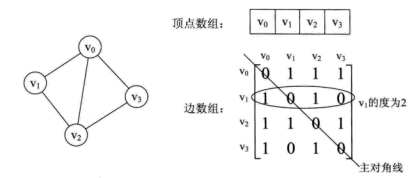邻接表表示的无向图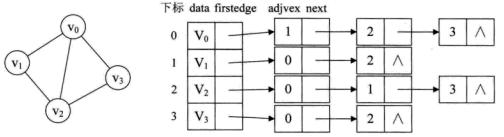1. 最短路径

Dijkstra

维护一个最短路径的的集合(sptSet)和最短距离数组, 直到遍历所有的点, 初始化起始点的距离是0, 集合为空.
初始化起始点s到所有的点的距离是INF, 注意s到s的距离是0.
while sptSet 不包含所有的顶点:

选择当前能到达点的最小距离的点u,加入 sptSet
使用u作为中间顶点,更新所有点的距离,选择最小距离的替换
dist[u]+graph[u][v] < dist[v]

百度百科
wikipedia
int minDistance(vector<int> dist, set<int> sptSet) {
int min_d = INT_MAX, u;
for(int i = 0, i < dist.size(); i ++) {
if(sptSet.find(i) == sptSet.end() && dist[v] < min_d) {
min_d = dist[i], u = i;
}
}
return u;
}
// 使用vector 表示的邻接矩阵, return 起始点到所有点的最小距离
// 没有边的用0填充
vector<int> dijstra(vector<vector<int>> graph, set<int> &sptSet,int src) {
int V = graph.size();
vector<int> dist(V, 0);
for(int i = 0;i < V; i ++) {
if(i != src) dist[i] = INT_MAX;
}
while(sptSet.size() < V-1) {
// pick mininum distance u
int u = minDistance(dist, sptSet);
sptSet.insert(u);
// 使用u更新距离
for(int v = 0; v < V; v ++) {
if(sptSet.find(v)==sptSet.end() && graph[u][v]
&& dist[u] != INT_MAX
&& dist[u]+graph[u][v] < dist[v]) {
dist[v] = dist[u] + graph[u][v];
}
}
}
return dist;
}



Floyd Warshall
Floyd算法是使用动态规划算法, dist[i][j]表示i–>j的最短距离,
那么是否存在i–>k–>j的路径小于dist[i][j],于是就有了下面的更新公式,
if dist[i][k] + dist[k][j] < dist[i][j]:
dist[i][j] = dist[i][k] + dist[k][j]

百度百科
wikipedia
void floydWarshall(vector<vector<int> > graph, vector<vector<int>> &dist, vector<vector<int> > &path) {
int V = graph.size();
// 参数dist和path需要初始化大小, 确定是否已经初始化
vector<vector<int> > tmp(V, vector<int>(V));
dist = path = tmp;
for(int i = 0; i < V; i ++) {
for(int j = 0; j < V; j ++) {
dist[i][j] = graph[i][j];
path[i][j] = j;
}
}
for(int i = 0; i < V; i++) {
for(int j = 0; j < V; j++) {
for(int k = 0; k < V; k++) {
if(dist[i][j] > dist[i][k] + dist[k][j]) {
dist[i][j] = dist[i][k] + dist[k][j];
pre[i][j] = pre[i][k];
}
}
}
}
}
//打印最短路径 u ---> v
int pfpath(int u, int v, vector<vector<int> > path) {
while(u != v) {
cout << u  << " ";
u = path[u][v];
}
cout << u << endl;
}

2. 最小支撑树

Prim Algorithm

用一个集合mstSet维护已经满足要求的顶点
使用dist表示从mstSet集合某个点到u的最小距离为INF, 初始点Src的距离是0.
while mstSet doesn’t include all vertices:

选择一个不在mstSet中, 并且在dist中距离最小的顶点u, 加入到mstSet
使用u更新dist距离, 表示从mstSet某个点到达为使用的点的最小距离

百度百科
wikipedia
int minDistance(vector<int> dist, set<int> mstSet) {
int min_d = INT_MAX, u;
for(int i = 0, i < dist.size(); i ++) {
if(mstSet.find(i) == mstSet.end() && dist[v] < min_d) {
min_d = dist[i], u = i;
}
}
return u;
}
// 使用vector 表示的邻接矩阵, return 起始点到所有点的最小距离
// 没有边的用0填充
vector<int> dijstra(vector<vector<int>> graph, set<int> &mstSet,int src) {
int V = graph.size();
vector<int> dist(V, 0);
int parent[V]; // 每个顶点的相邻的点
parent[src] = -1;
for(int i = 0;i < V; i ++) {
if(i != src) dist[i] = INT_MAX;
}
while(mstSet.size() < V-1) {
// pick mininum distance u
int u = minDistance(dist, sptSet);
mstSet.insert(u);
// 使用u更新距离
for(int v = 0; v < V; v ++) {
if(mstSet.find(v)==mstSet.end() && graph[u][v]
&& graph[u][v] < dist[v]) {
dist[v] = graph[u][v];
parent[v] = u;
}
}
}
return dist;
}



Kruskal Algorithm

根据权重排序所有的边
选择一个小权重的边,如果它没有和最小支撑顶点形成环,就加入这个边
重复2,知道包含V-1个边

百度百科
wikipedia
Code 抄写
struct Edge {
int src, dest, weight;
};
struct Graph {
int V, E;
struct Edge* edge;
};
struct Graph* createGraph(int V, int E) {
struct Graph* graph = new Graph;
graph->V = V;
graph->E = E;
graph->edge = new Edge[E];
return graph;
}
struct subset {
int parent;
int rank;
};
int find(struct subset subsets[], int i) {
if (subsets[i].parent != i)
subsets[i].parent = find(subsets, subsets[i].parent);
return subsets[i].parent;
}
void Union(struct subset subsets[], int x, int y) {
int xroot = find(subsets, x);
int yroot = find(subsets, y);
if (subsets[xroot].rank < subsets[yroot].rank)
subsets[xroot].parent = yroot;
else if (subsets[xroot].rank > subsets[yroot].rank)
subsets[yroot].parent = xroot;
else {
subsets[yroot].parent = xroot;
subsets[xroot].rank++;
}
}
int myComp(const void* a, const void* b) {
struct Edge* a1 = (struct Edge*)a;
struct Edge* b1 = (struct Edge*)b;
return a1->weight > b1->weight;
}
void KruskalMST(struct Graph* graph) {
int V = graph->V;
struct Edge result[V];
int e = 0;
int i = 0;
qsort(graph->edge, graph->E, sizeof(graph->edge), myComp);
struct subset *subsets =
(struct subset*) malloc( V * sizeof(struct subset) );
for (int v = 0; v < V; ++v) {
subsets[v].parent = v;
subsets[v].rank = 0;
}
while (e < V - 1) {
struct Edge next_edge = graph->edge[i++];
int x = find(subsets, next_edge.src);
int y = find(subsets, next_edge.dest);
if (x != y) {
result[e++] = next_edge;
Union(subsets, x, y);
}
}
return;
}

3. 拓扑排序
定义: 对一个有向无环图(Directed Acyclic Graph简称DAG)G进行拓扑排序，是将G中所有顶点排成一个线性序列，使得图中任意一对顶点u和v，若边(u,v)∈E(G)，则u在线性序列中出现在v之前。

计算所有节点的入度
每次选择一个入度为0的顶点u,如果的已经排序的结果中
将u所到达的所有顶点v,入度减1,
重复1,2,直到遍历所有顶点

百度百科
wikipedia
class Graph {
int V;  // 顶点的个数
};

void topologicalSort(int V, list<int> *adj) {
// 计算所有入度
vector<int> in_degree(V, 0);
for (int u=0; u<V; u++) {
list<int>::iterator itr;
in_degree[*itr]++;
}
}
// 加入入度为0的点
queue<int> q;
for (int i = 0; i < V; i++) {
if (in_degree[i] == 0) q.push(i);
}
int cnt = 0;
vector <int> top_order;
while (!q.empty()) {
int u = q.front();
q.pop();
top_order.push_back(u);
// 所有连接点, 入度减去1
list<int>::iterator itr;
if (--in_degree[*itr] == 0) q.push(*itr);
}
cnt++;
}
if (cnt != V) {
cout << "There exists a cycle in the graph\n";
return;
}
for (int i=0; i<top_order.size(); i++)
cout << top_order[i] << " ";
cout << endl;
}

4. 有向图判环
题目: 请你判断一个 n 个点，m 条边的有向图是否存在环。参数为两个int数组，start[i]到end[i]有一条有向边.
解析: 这是拓扑排序的一种应用.
bool isCyclicGraph(vector<int> &start, vector<int> &end) {
// 找到最大顶点值,构造图,
int n = 0;
for (int s : start) {
n = max(n, s);
}
for (int e : end) {
n = max(n, e);
}
// 构造图
vector<vector<int>> graph(n + 1);
vector<int> d(n + 1);
int m = start.size();
// 计算所有顶点的入度
for (int i = 0; i < m; i++) {
graph[start[i]].push_back(end[i]);
d[end[i]]++;
}
queue<int> que;
// 将所有入度为0的点,加入队列
for (int i = 1; i <= n; i++) {
if (graph[i].size() && !d[i]) {
que.push(i);
}
}
while (!que.empty()) {
int h = que.front();
que.pop();
// 将多有入度为0的点,对应的顶点 入度减去1
for (int y : graph[h]) {
d[y]--;
if (!d[y]) {
que.push(y);
}
}
}
// 判断是否所有顶点的入度都是0, 如果是,则没有环,否则就有
for (int i = 1; i <= n; i++) {
if (d[i]) {
return true;
}
}
return false;
}

参考

https://www.cnblogs.com/Ash-ly/p/5920953.html
https://www.geeksforgeeks.org/graph-data-structure-and-algorithms/


展开全文• 图由顶点(vertex)和边(edge)组成，通常表示为G = (V, E) G标识一个图，V是顶点集，E是边集 顶点集V有穷且非空 ...有向图（Directed Graph） 有向图的边是有明确方向的 有向无环图（Directed Acycl...


图（Graph）- 遍历

图（Graph）- AOV网（Activity On Vertex Nework）- 拓扑排序（Topological Sort）

图（Graph）- 生成树（Spanning Tree）

图（Graph）- 生成树（Spanning Tree）- Prim（普里姆算法）

图（Graph）- 生成树（Spanning Tree）- Kruskal（克鲁斯科尔算法）

图（Graph）- 最短路径（Shortest Path）

图（Graph）- 最短路径（Shortest Path）- Dijkstra（迪杰斯特拉算法）

图（Graph）- 最短路径（Shortest Path）- Bellman-Ford（贝尔曼-福特算法）

图（Graph）- 最短路径（Shortest Path）- Floyd（弗洛伊德算法）

图（Graph）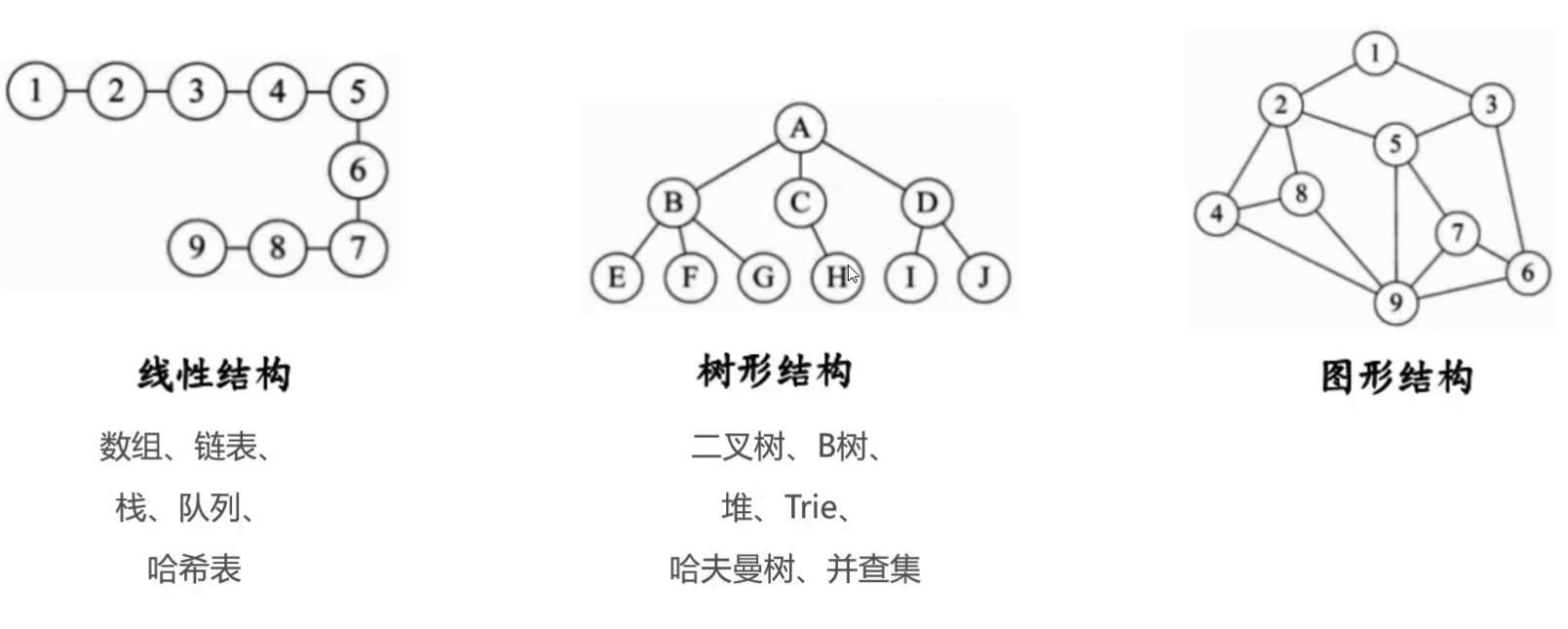图由顶点(vertex)和边(edge)组成，通常表示为G = (V, E)

G标识一个图，V是顶点集，E是边集
顶点集V有穷且非空
任意两个顶点之间都可以用边来表示它们之间的关系，边集E可以是为空的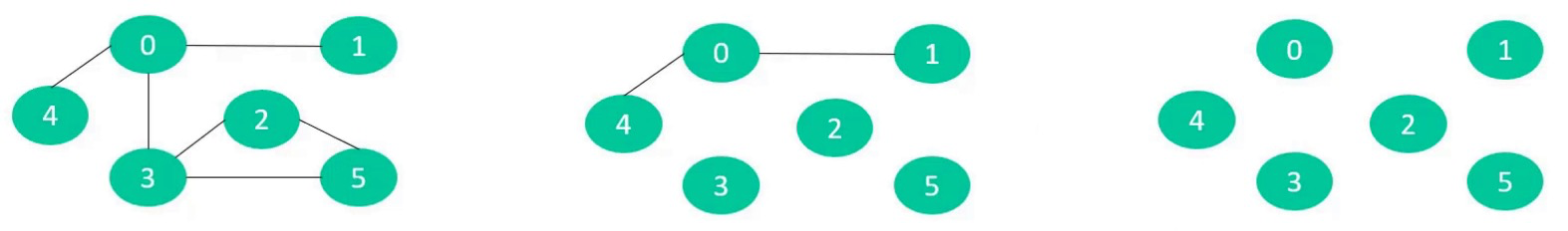图的应用举例

图结构的应用极其广泛

社交网络
地图
游戏开发
…有向图（Directed Graph）

有向图的边是有明确方向的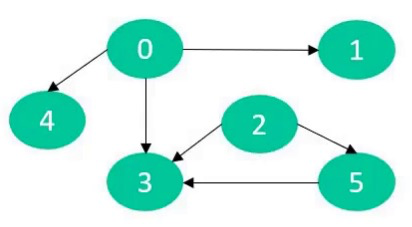有向无环图（Directed Acyclic Graph，简称DAG）
如果一个有向图，从任意顶点出发无法经过若干条边回到该顶点，那么它就是一个有向无环图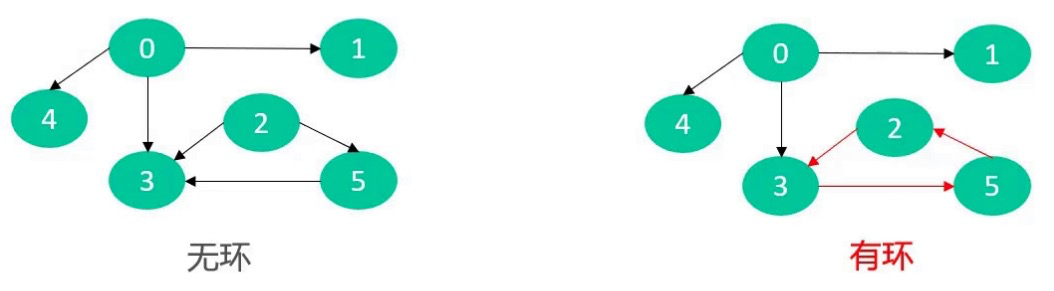出度、入度
出度、入度适用于有向图

出度（Out-degree）

一个顶点的出度为x，是指有x条边以该顶点为起点
顶点11的出度是3

入度（In-degree）

一个顶点的入度为x，是指有x条边以该顶点为终点
顶点11的入度是2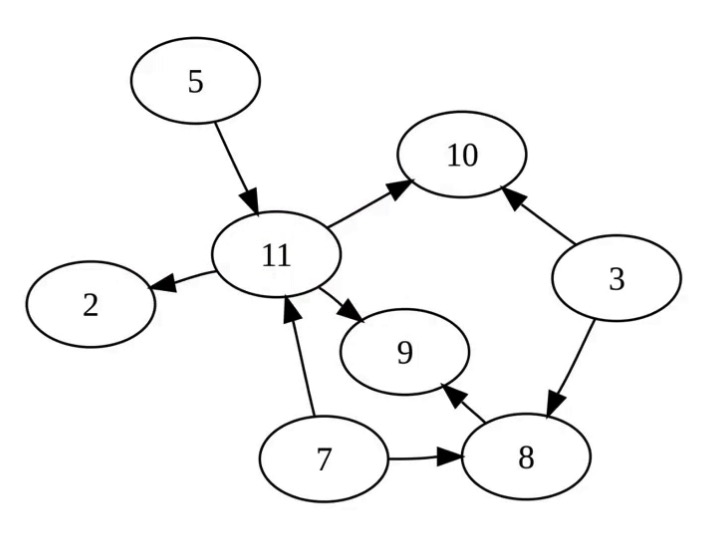无向图（Undirected Graph）

无向图的边是无方向的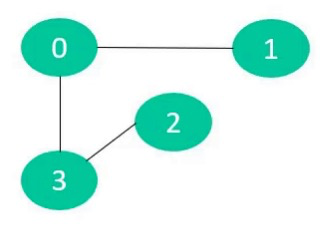效果类似于下面的有向图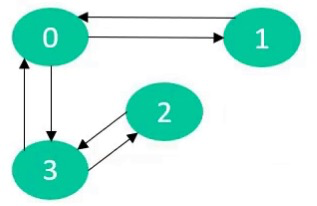混合图（Mixed Graph）

混合图的边可能是无向的，也可能是有向的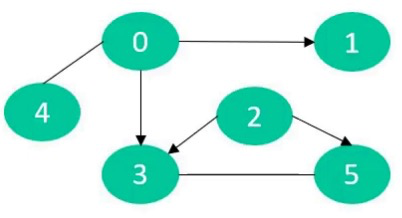简单图、多重图

平行边

无向图中，关联一对顶点的无向边如果多于1条，则称这些边为平行边
在有向图中，关联一对顶点的有向边如果多于1条，并且它们的方向相同，则称这些边为平行边

多重图（Multi Graph）
有平行边或者有自环的图
简单图（Simple Graph）
既没有平行边也没有自环的图
博客中讨论的基本都是简单图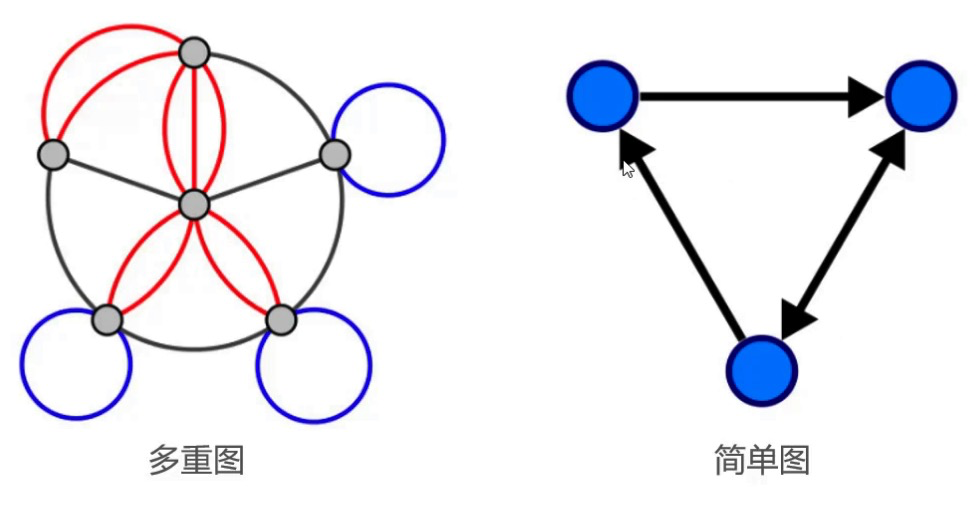无向完全图（ Undirected Complete Graph）

无向完全图的任意两个顶点之间都存在边
n个顶点的无向完全图有n (n - 1) / 2条边
(n - 1) + (n - 2) + (n - 3) + … + 3 + 2 + 1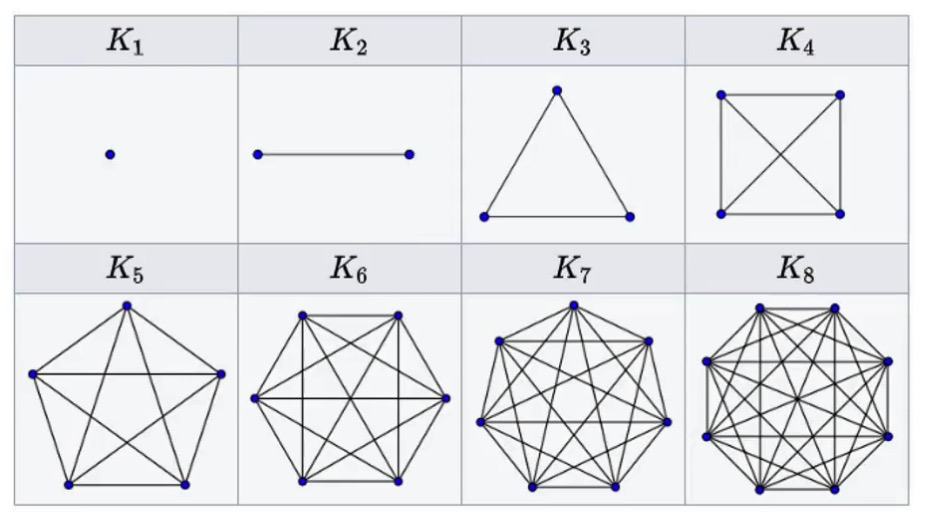有向完全图（ Directed Complete Graph）

有向完全图的任意两个顶点之间都存在方向相反的两条边
n个顶点的有向完全图有n(n-1)条边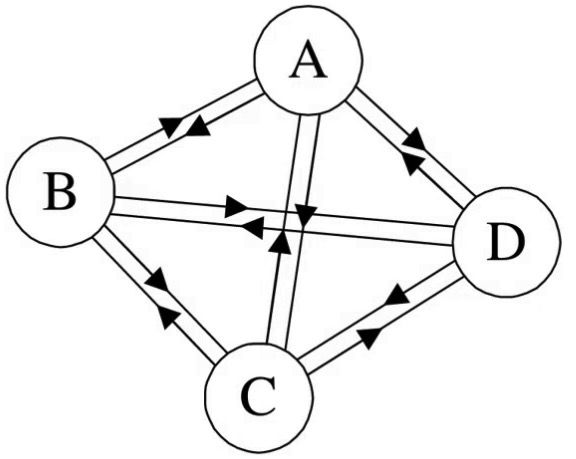稠密图（Dense Graph）：边数接近于或等于完全图
疏密秃（Sparse Graph）：边数远远少于完全图

有权图（ Weighted Graph）

有权图的边可以拥有权值（Weight）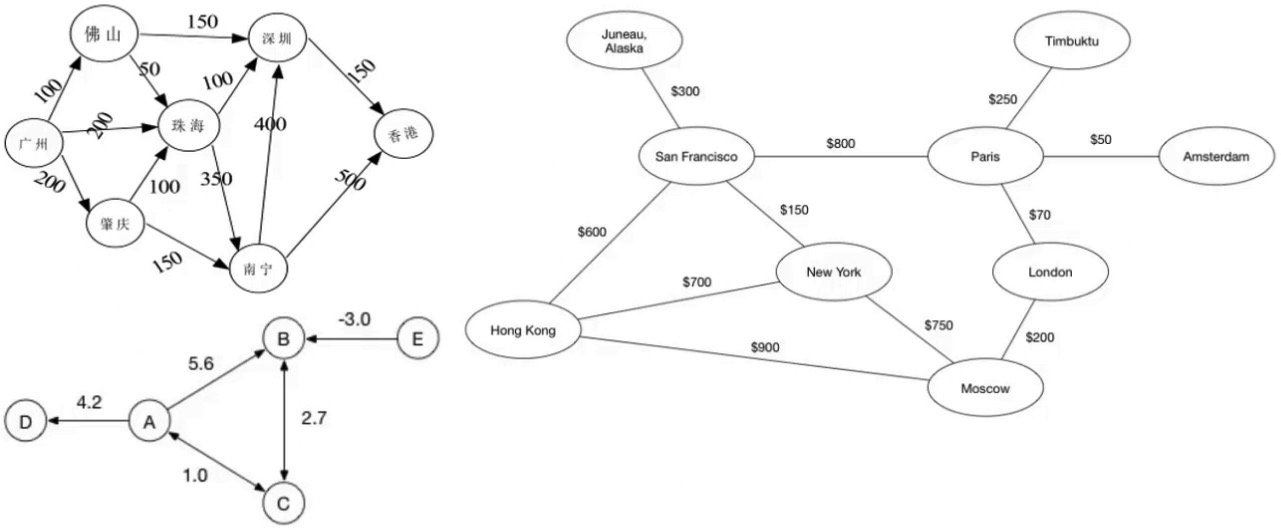连通图（ Connected Graph）

如果顶点x和y之间存在可相互抵达的路径（直接或者间接的路径），则称x和y是连通的
如果无向图G中任意2个顶点都是连通的，则称G为连通图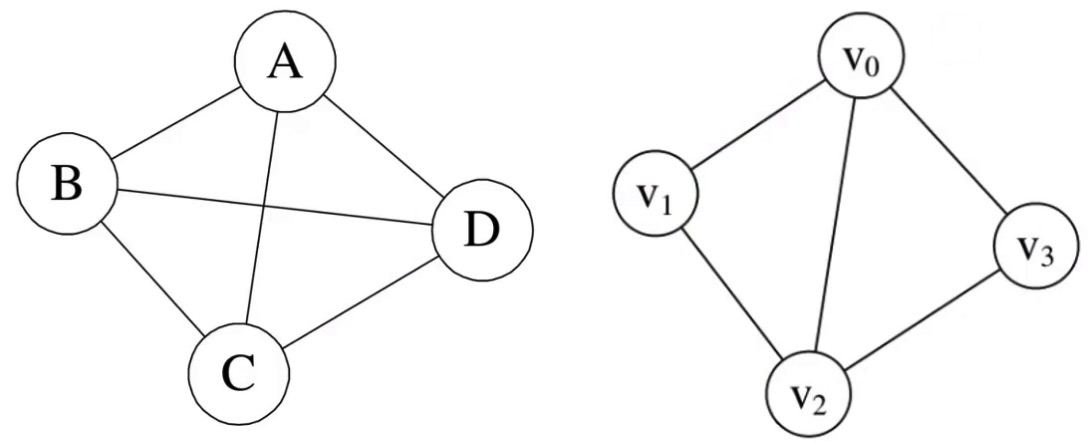连通分量（ Connected Component）

如果顶点x和y之间存在可相互抵达的路径（直接或者间接的路径），则称x和y是连通的
连通图只有一个连通分量，即其自身；非连通的无向图有多个连通分量
下面的无向图有3个连通分量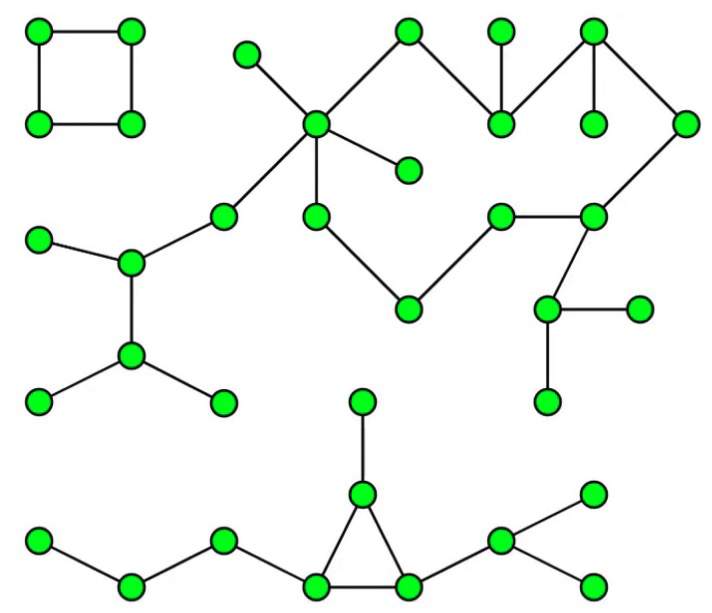强连通图（ Strongly Connected Graph）

如果有向图G中任意2个顶点都是连通的，则称G为强连通图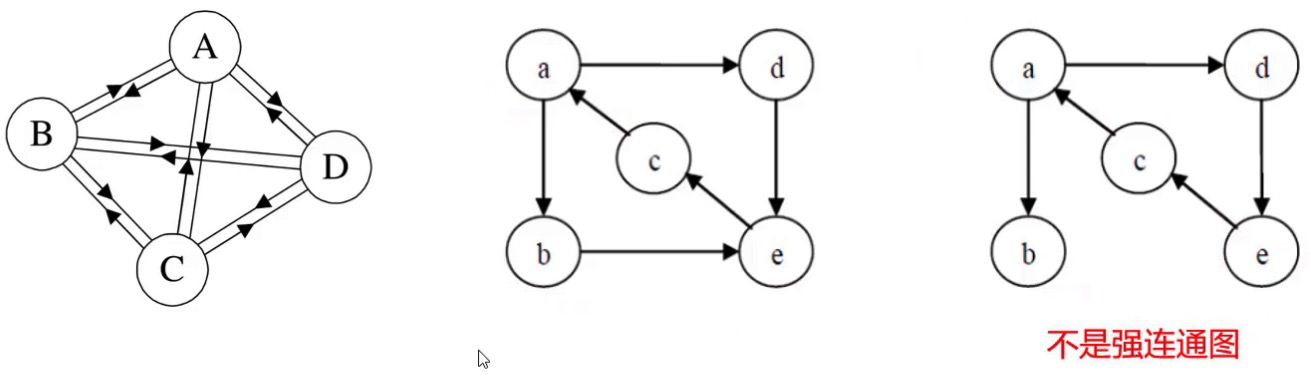强连通分量（ Strongly Connected Component）

强连通分量：有向图的极大强连通子图
强连通图只有一个强连通分量，即其自身；非强连通的有向图有多个强连通分量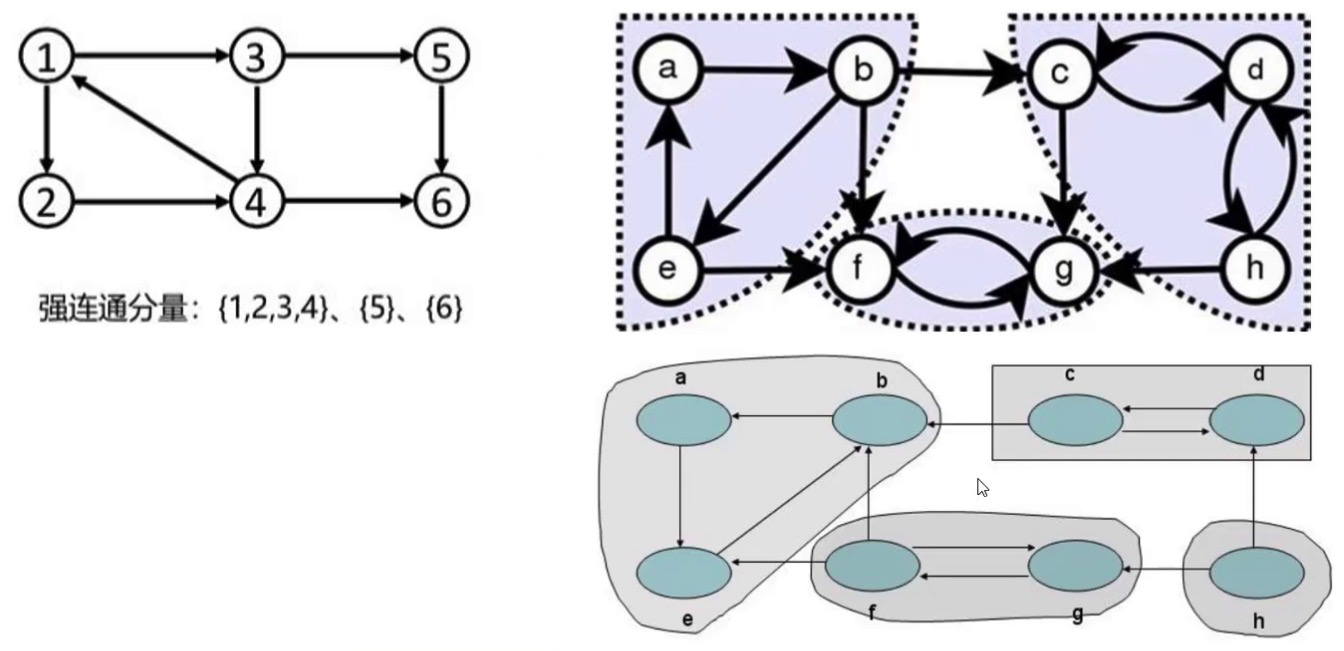图的实现方案

图由2种常见的实现方案

邻接矩阵的存储方式

一位数组存放顶点信息
二位数组存放边信息

邻接矩阵比较适合稠密图
不然会比较浪费内存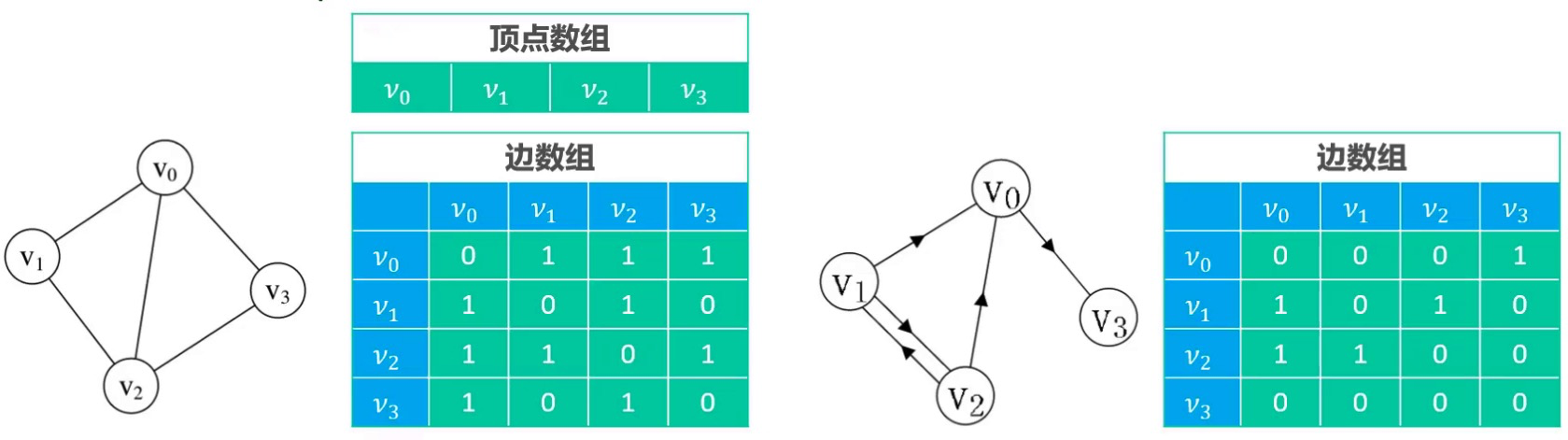邻接矩阵 - 有权图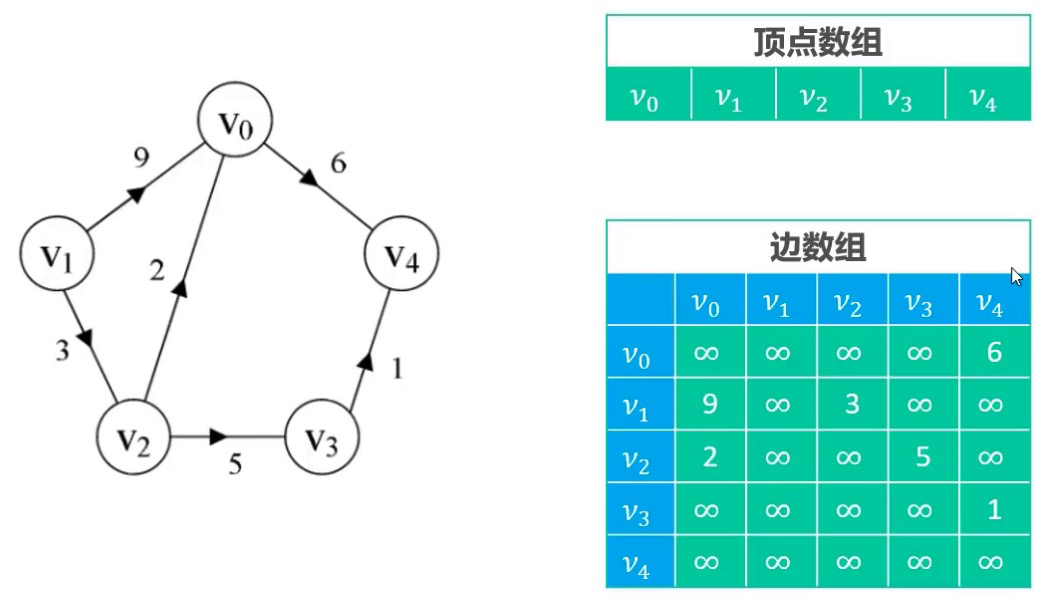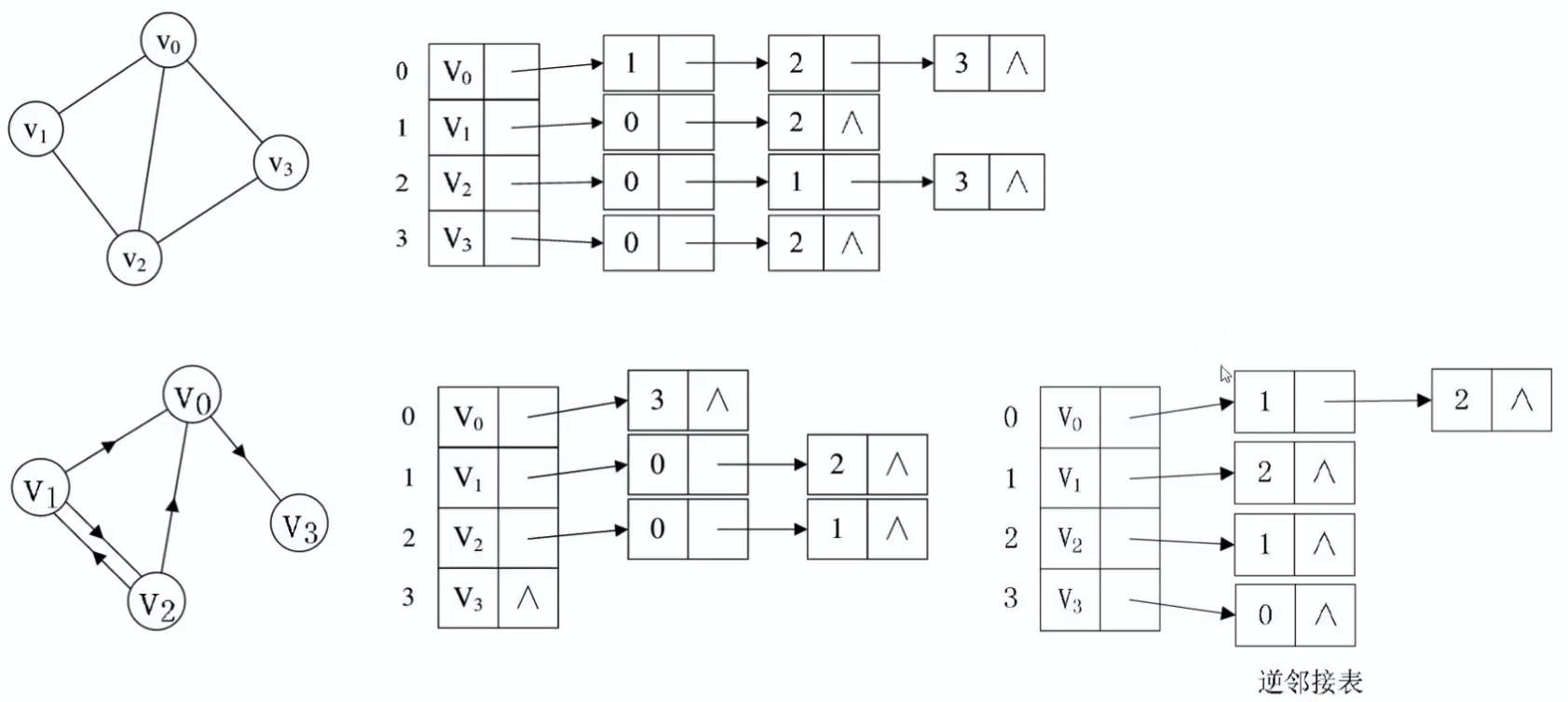邻接表- 有权图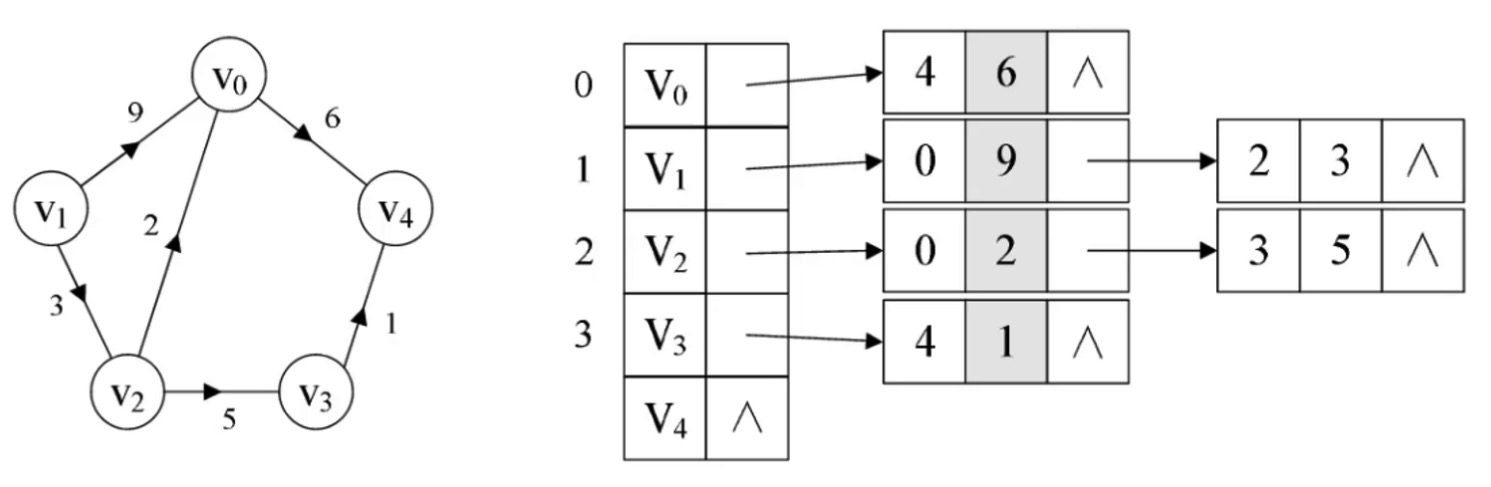图的基础接口
int verticesSize();
int edgesSize();

void removeVertex(V v);

void addEdge(V fromV, V toV, E weight);
void removeEdge(V fromV, V toV);

顶点的定义
private static class Vertex<V, E> {
V value;
Set<Edge<V, E>> inEdges = new HashSet<>();
Set<Edge<V, E>> outEdges = new HashSet<>();
Vertex(V value) {
this.value = value;
}
@override
public boolean equals(Object obj) {
return Objects.eqauls(value, ((Vertex<V, E> obj).value));
}
@override
public int hashCode() {
return value == null ? 0 : value.hashCode();
}
}

边的定义
private static class Edge<V, E> {
Vertex<V, E> from;
Vertex<V, E> to;
E weight;
public boolean equals(Object obj) {
Edge<V, E> edge = (Edge<V, E>) obj;
return from.equals(edge.from) && to.equal(edge.to);
}
public int hashCode() {
return form.hashCode() * 31 + to.hashCode();
}
}



展开全文• 图的基本定义 图（Graph）是由顶点的又穷非空集合和顶点之间边的集合组成，通常表示为：G(V,E)，其中，G表示一个图，V是图G中顶点的集合，E是图G中变得集合。介绍了各种不同的图，有向图和无向图，主要区别是点的...
图的定义

图（Graph）是由顶点的又穷非空集合和顶点之间边的集合组成，通常表示为：G(V,E)，其中，G表示一个图，V是图G中顶点的集合，E是图G中变得集合。

需要注意的是：数据元素在线性表中叫元素，树中叫结点，在图中称之为顶点(Vertex)；在图结构中，不允许没有顶点，在定义中，若V为顶点集合，则强调了顶点集合V有穷非空；在图中，任意两个顶点之间都可能有关系，顶点间的逻辑关系用边来表示，边集可为空。

各种图的定义

无向边：若顶点 vi<!--//--><![CDATA[//><!--
v_i
//--><!]]> 到 vj<!--//--><![CDATA[//><!--
v_j
//--><!]]> 之间的边没有方向，则称这条边为无向边(Edge)，用无需偶对（vi<!--//--><![CDATA[//><!--
v_i
//--><!]]>，vj<!--//--><![CDATA[//><!--
v_j
//--><!]]>）来表示。若图中任意两个顶点之间的边为无向边，则称该图为无向图（Undirected graphs）。

有向边：若从顶点 vi<!--//--><![CDATA[//><!--
v_i
//--><!]]> 到 vj<!--//--><![CDATA[//><!--
v_j
//--><!]]> 的边有方向，则称这条边为有向边，也称为弧(Arc)。用有序偶<vi<!--//--><![CDATA[//><!--
v_i
//--><!]]>，vj<!--//--><![CDATA[//><!--
v_j
//--><!]]>>，vi<!--//--><![CDATA[//><!--
v_i
//--><!]]> 称为弧尾（Tail），vj<!--//--><![CDATA[//><!--
v_j

注意：无向边用小括号“（）”，而有向边则是用尖括号“<>”表示。

在图中，若不存在顶点到其自身的边，且同一条边不重复出现，则称这样的图为简单图。

在无向图中，如果任意两个顶点之间都存在边，则称该图为无向完全图。含有n个顶点的无向完全图有 n∗(n−1)/2<!--//--><![CDATA[//><!--
n*(n-1)/2
//--><!]]> 条边。

在有向图中，如果任意两个顶点之间都存在方向弧尾相反的两条弧，则称该图为有向完全图。含有n个顶点的有向完全图有 n∗(n−1)<!--//--><![CDATA[//><!--
n*(n-1)
//--><!]]> 条边。

有很少条边或弧的图称为稀疏图，反之称为稠密图。

与图的边或弧相关的数叫做权（Weight）。将带权的图通称为网（NetWork）。

假设有两个图G=（V，{E}）和G’=（V’，{E’}），如果V′⊆V<!--//--><![CDATA[//><!--
V' \subseteq V
//--><!]]> 且E′⊆E<!--//--><![CDATA[//><!--
E' \subseteq E
//--><!]]>，则称G’为G的子图（SubGraphs）。

图的顶点与边间的关系

对于无向图G<!--//--><![CDATA[//><!--
G
//--><!]]>=(V<!--//--><![CDATA[//><!--
V
//--><!]]>，{E<!--//--><![CDATA[//><!--
E
//--><!]]>})，如果边 (v,v′)∈E<!--//--><![CDATA[//><!--
(v,v') \in E
//--><!]]>，则称顶点 v<!--//--><![CDATA[//><!--
v
//--><!]]> 和v′<!--//--><![CDATA[//><!--
v'
v
//--><!]]> 和 v′<!--//--><![CDATA[//><!--
v'
//--><!]]> 相邻接。边 (v,v′)<!--//--><![CDATA[//><!--
(v,v')
//--><!]]> 依附于顶点 v<!--//--><![CDATA[//><!--
v
//--><!]]> 和 v′<!--//--><![CDATA[//><!--
v'
//--><!]]> ，或者说 (v,v′)<!--//--><![CDATA[//><!--
(v,v')
//--><!]]> 与顶点 v<!--//--><![CDATA[//><!--
v
//--><!]]> 和 v′<!--//--><![CDATA[//><!--
v'
//--><!]]> 相关联。顶点 v<!--//--><![CDATA[//><!--
v
//--><!]]> 的度是和 v<!--//--><![CDATA[//><!--
v
//--><!]]> 相关联的边的数目。

对于有向图G<!--//--><![CDATA[//><!--
G
//--><!]]>=(V<!--//--><![CDATA[//><!--
V
//--><!]]>，{E<!--//--><![CDATA[//><!--
E
//--><!]]>})，如果弧 <v,v′>∈E<!--//--><![CDATA[//><!--
<v,v'>\in E
//--><!]]>，则称顶点 v<!--//--><![CDATA[//><!--
v
//--><!]]>邻接到顶点 v′<!--//--><![CDATA[//><!--
v'
//--><!]]> ，顶点 v′<!--//--><![CDATA[//><!--
v'
//--><!]]>邻接自顶点 v<!--//--><![CDATA[//><!--
v
//--><!]]> 。弧 <v,v′><!--//--><![CDATA[//><!--
<v,v'>
//--><!]]> 和顶点 v<!--//--><![CDATA[//><!--
v
//--><!]]> 和 v′<!--//--><![CDATA[//><!--
v'
//--><!]]> 相关联。以顶点 v<!--//--><![CDATA[//><!--
v
//--><!]]> 为头的弧的数目称为 v<!--//--><![CDATA[//><!--
v
//--><!]]> 的入度（InDegree），记为ID（v<!--//--><![CDATA[//><!--
v
//--><!]]>）；以 v<!--//--><![CDATA[//><!--
v
//--><!]]> 为尾的弧的数目称为 v<!--//--><![CDATA[//><!--
v
//--><!]]> 的出度（OutDegree），记为OD（ v<!--//--><![CDATA[//><!--
v
//--><!]]>），顶点 v<!--//--><![CDATA[//><!--
v
//--><!]]> 的度为出度和入度之和。

无向图从顶点 v<!--//--><![CDATA[//><!--
v
//--><!]]> 到顶点 v′<!--//--><![CDATA[//><!--
v'
//--><!]]> 的路径（path）是一个顶点序列。路径的长素是路径的边或弧的数目。

第一个顶点到最后一个顶点相同的路径称为回路或环（Cycle）。序列中 顶点不重复出现的路径称为简单路径。除了第一个顶点和最后一个顶点之外，其与定点不重复出现的回路，成为简单回路或简单环。

连通图

在无向图G中，如果顶点 v<!--//--><![CDATA[//><!--
v
//--><!]]> 到顶点 v′<!--//--><![CDATA[//><!--
v'
//--><!]]> 有路径，则称 v<!--//--><![CDATA[//><!--
v
//--><!]]> 和 v′<!--//--><![CDATA[//><!--
v'
//--><!]]> 是连通的。如果对于图中任意两个顶点 vi,vj∈V<!--//--><![CDATA[//><!--
v_i,v_j \in V
//--><!]]> ，vi<!--//--><![CDATA[//><!--
v_i
//--><!]]> 和 vj<!--//--><![CDATA[//><!--
v_j
//--><!]]>都是连通的，则称G为连通图（Connected Graphs）。

无向图中的极大连通子图称为连通分量，强调以下几点：是子图；子图要连通；连通子图含有极大顶点数；具有极大定点的连通子图包含依附于这些顶点的所有边。

在有向图G中，如果对于每一对 vi,vj∈V,vi≠vj<!--//--><![CDATA[//><!--
v_i,v_j \in V,v_i \neq v_j
//--><!]]> ，从vi<!--//--><![CDATA[//><!--
v_i
//--><!]]> 到 vj<!--//--><![CDATA[//><!--
v_j
//--><!]]> 和从 vj<!--//--><![CDATA[//><!--
v_j
//--><!]]> 到 vi<!--//--><![CDATA[//><!--
v_i
//--><!]]> 都存在路径，则称G是强连通图。有向图中的极大强连通子图称做有向图的强连通分量。

一个连通图的生成树是一个极小的连通子图，它包含图中全部的n个顶点，但只有足以构成一棵树的n-1条边。

若一个有向图恰有一个顶点的入度为0，其余顶点的入度均为1，则是一棵有向树。一个有向图的生成森林有若干棵有向树组成，含有图中全部顶点，但只有足以构成若干棵树不相交的有向树的弧。
展开全文Graph
• 前言：利用图类数据结构，可以运用到GPS，google地图等需要计算最短路径的算fa中。 1，一个图G = (V, E)由以下元素组成。  V：一组顶点  E：一组边，连接V中的顶点  2，图的表示：邻接矩阵，邻接表，关联...
• 图是由顶点(vertex)集合及顶点间的关系组成的一种数据结构。 本文主要介绍图的定义以及图的相关术语。数据结构
•graph 数据结构 基础
• Graph 图是一种非线性表结构, 用来模拟一组连接 图的算法有很多, 比如图的搜索、最短路径、最小生成树、二分图等 概念 顶点（vertex）:图中的元素 边（edge）:顶点之间建立的连接关系 无向图: 边没有方向的图, ...广度优先算法 深度优先算法 N皇后算法
• 《Relational inductive biases, deep learning, and graph networks》 这篇论文包含了一部分新研究、一部分回顾和部分统一结论，这篇文章涉及到的很多知识面，涉及到联结主义、行为主义、符号主义，其本身的模型并...
• 导入keras的时候出现了版本不兼容的情况。 我的环境： windows10 python3.5.6 tensorflow-gpu1.10.0 在使用pip install keras 默认版本安装完成后，使用 import keras ...Traceback (m...
• 前言 分析社会关系这类复杂图壮结构的海量数据，使用图形数据库（Graph DataBase）是最好的选择。
• 图像分割之（二）Graph Cut（图割） zouxy09@qq.com http://blog.csdn.net/zouxy09    上一文对主要的分割方法做了一个概述。那下面我们对其中几个比较感兴趣的算法做个学习。下面主要是Graph Cut，下一个博文...
• 软件环境 Unity Version: 2018.1.2f1 边缘发光材质效果 创建工程 打开Unity并创建一个新工程 ... Package Manager打开包管理器，安装二个依赖包： ...2. Shader Graph Lightweight Render Pipeline ...Unity Shader
• 大智和你一起学习ShaderGraph，在实战中探索。 课程内容 ShaderGraph的基本使用 丰富的实战案例： · 全息效果 · 边缘光效果 · 溶解效果 ·&...unity3d unity3d下载 unity3d安装 unity3d小游戏
• 图（Graph）是一个常见的数据结构，现实世界中有很多很多任务可以抽象成图问题，比如社交网络，蛋白体结构，交通路网数据，以及很火的知识图谱等，甚至规则网络结构数据（如图像，视频等）也是图数据的一种特殊形式...Deepwalk Line node2vec
• 当我刚接触Elastic的Graph时，我对Graph的理解确实是模糊的。从字面上讲，它的意思是“图形”的意思。那个它在Elasticsearch中到底代表是什么？经过一段时间的探索，我对这个Graph有一些初步的认识。简单地说：graph...Elastic
• 图（graph）是一种数据格式，它可以用于表示社交网络、通信网络、蛋白分子网络等，图中的节点表示网络中的个体，连边表示个体之间的连接关系。许多机器学习任务例如社团发现、链路预测等都需要用到图结构数据，因此...图卷积神经网络
• 1.GraphMaker只针对unity的UGUI开发，NGUI要用GraphMaker_NGUI， 可接入第三方插件。 2.生成图表对象要通过GraphMaker内部封装的函数生成，并且要在Start()函数里生成，生成、赋值、设父节点后要立刻调用 Init() ...图表
• 利用泛型实现一个Graph类 一、 定义一个接口Graph.java： public interface Graph&lt;L&gt; { public static &lt;L&gt; Graph&lt;L&gt; empty() { } //其中这个方法需要具体实现 ### ...ADT generic Graph
• 之前讲完变量常量等等基本量的操作，意味着...这些就是计算图谱graph和Session的作用了。IV.Graphhttps://www.tensorflow.org/versions/r0.11/api_docs/python/framework.html#Graph 一个TensorFlow的运算，被表示为一tensorflow python
...